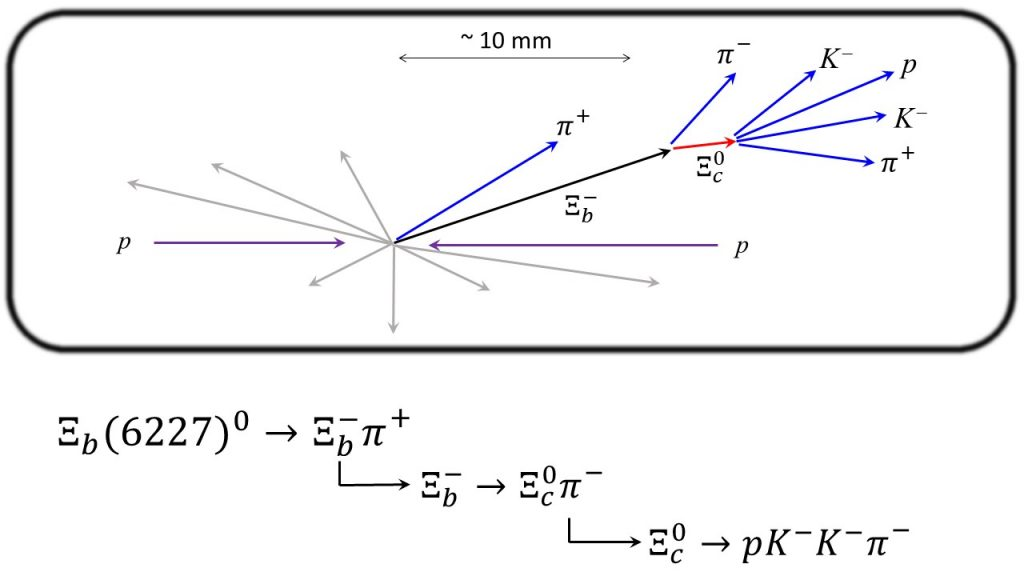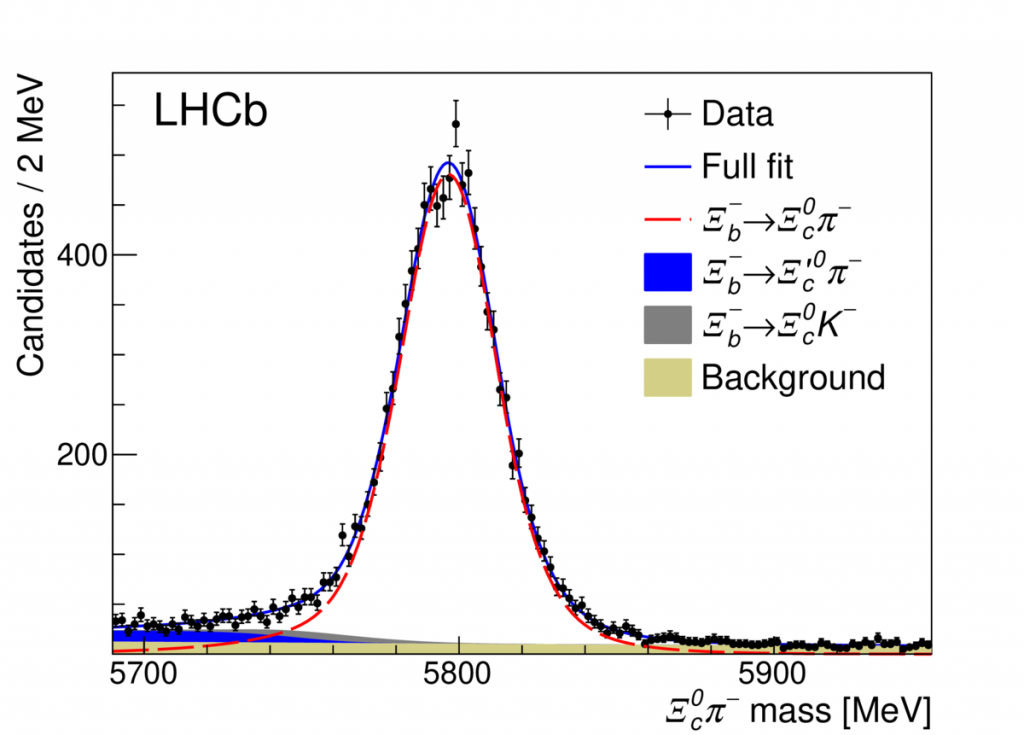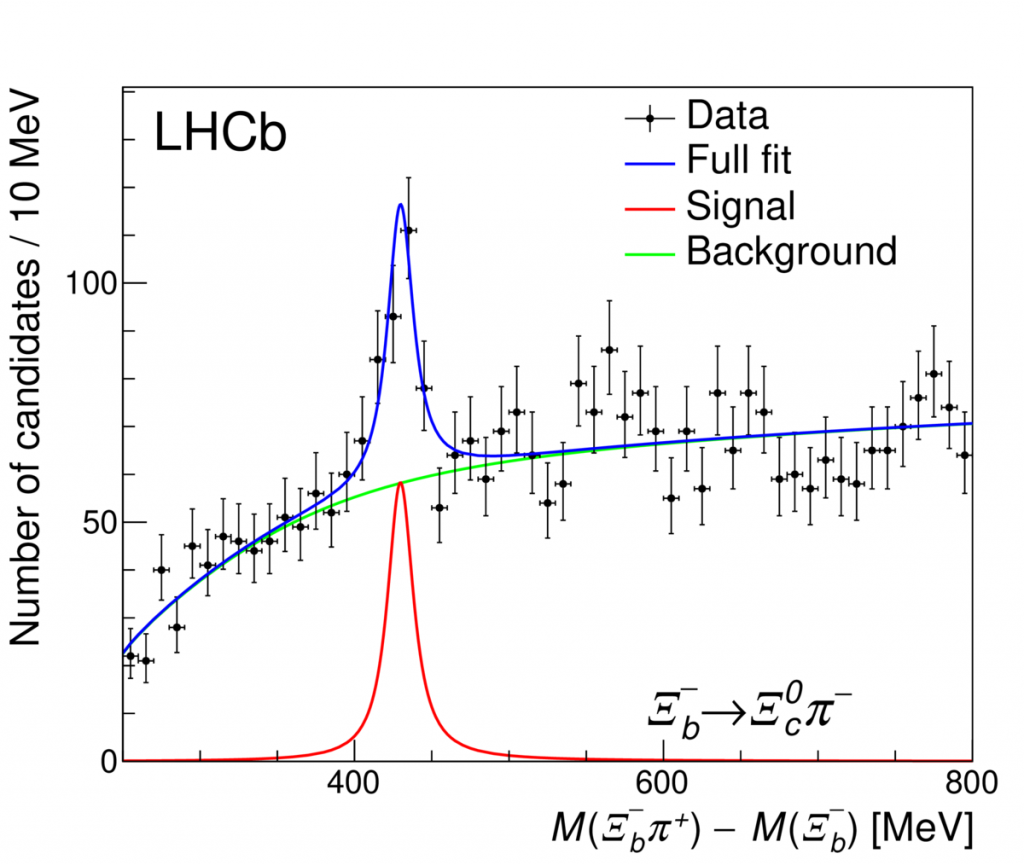# Observation of a New Ξb0 State

In pp collisions at LHCb, a very large number of hadrons containing beauty quarks are produced. These hadrons include the ground state mesons — B0 (bd), B+ (bu) and Bs (bs) — but also beauty baryons. The weakly-decaying ground state baryons that include one b quark and either u, d or s quarks are the Λb0 (bud),  Ξb0 (bsu), Ξb (bsd), and Ωb (bss). In each case, we show the quark content according to the quark model.

Quantum mechanics predicts that the bound state of a system of particles should produce distinct states, each characterized by “good” quantum numbers. For Hydrogen, the electron can exist in the 1s, 2p, 3d, etc, quantum states, where the number refers to the principal quantum number, e.g. n, associated with the radial motion, and the letters (s, p, d) refer to the orbital angular momentum, L = 0, 1, 2, respectively, in units of ℏ. The energies of the bound states of Hydrogen are sensitive to the the nature of the electromagnetic force. Since the principal energy levels of Hydrogen are En = -13.6 eV / n2 , the energy-level splittings are at the level of a few electronvolts. Much finer splitting occurs between states with the same n value, but different orbital and/or spin angular momenta. To predict precisely the finest energy level splittings of Hydrogen (e.g Lamb Shift), one must employ the quantum theory of electromagnetism, namely Quantum Electrodynamics.

In a similar way, by studying the spectrum of bound states of quarks, we can learn about the theory of the strong force, or Quantum Chromodynamics. Because the strong force is strong, the energy-level splittings are not eV, but millions of electronvolts, or MeV. Because of these large energy-level splittings, one arrives at distinct masses (m = E / c2) among these states of different quantum numbers. The excited states are therefore considered distinct particles, bound by the strong force.

In this analysis, we search for excited Ξb0 states. Evidence is sought by choosing a specific manner (decay mode) in which we expect such states to decay. An excited Ξb0 state would have quark content bus. The excited states are expected to decay through the strong interaction, in which case the decay can be mediated by emission of a gluon that then transforms into (say) a uu or dd pair. Let’s consider the latter case, where the final state now consists of  busdd. In the decay process, these 5 quarks could assemble as bsd + ud, which could manifest itself as Ξb π+. For the uu case, we could end up with Ξb0 π0. However, π0 mesons decay to two photons, and low energy photons have low detection efficiency in LHCb; therefore Ξb0 π0 is unlikely to be a good decay mode to search for excited Ξb0 states.

Below, we show the topology for the decay of an excited Ξb0 state, here referred to as Ξb(6227)0. To reconstruct a  Ξb(6227)0 candidate, we “work backwards” in terms of the decay sequence.If/when the state is produced, it is found by first reconstructing a Ξc0 → p KKΠ+ baryon candidate. The p KKΠ+ particles are required to converge to a single point in 3D space (within the detector resolution), and have an invariant mass consistent with the known Ξc0 mass of 2470 MeV/c2. Then, this Ξc0 candidate is combined with a Π meson to search for Ξb baryon candidates. The Ξc0 Π  combination is required to have an invariant mass consistent with the known Ξb mass of 5797 MeV/c2. Note that all of the particles used to form the Ξc0 and Ξb candidates must be inconsistent with coming directly from the proton-proton collision point. This is a common requirement, which exploits the large lifetime of beauty and charm hadrons, which results in them traveling several mm’s before decaying. In this case. the Ξb and Ξc0 lifetimes are about 1.6 ps and 0.15 ps, respectively (here, ps = 10-15 sec). The invariant mass distribution of Ξc0Π candidates passing all selection requirements is shown below. A prominent peak is seen at the expected Ξb mass. A fit to the data is overlaid.Lastly, the Ξb candidate is combined with a Π meson that is consistent with coming from the pp interaction point. Here, the Π is taken from the pp interaction point because the decay is expected to be a strong decay, and the decay time is very small, of order 10-23 sec.

Rather than use the invariant mass of the ΞbΠ+ candidate to search for the excited Ξb0 state, we use the difference in invariant mass, M(ΞbΠ+) – M(Ξb), where in both cases, we are referring to the invariant mass of the decay products. The advantage of doing this is that the dominant component contributing to the mass resolution — namely that coming from the Ξb invariant mass determination — cancels in this difference. With this difference, the mass resolution is primarily due to the momentum resolution of the single, low-momentum Π+ , which is very good.
The resulting mass difference spectrum is shown below.A very significant peak is observed at a mass difference value of 430 MeV/c2. Adding the known Ξb mass (5797 MeV/c2) to this peak value results in a measured peak mass of 6227.1 ± 1.5 MeV/c2. This peak represents the observation of a new state, likely to be an excited Ξb0 state. We have named it Ξb(6227)0

In the publication, an additional decay mode of the Ξb baryon was used, namely
Ξb →   Ξc0ΠΠ+Π. Also, improved measurements of the previously-observed charged Ξb(6227) state were reported using Ξb(6227) → Λb0K candidates.

More details, and additional measurements performed in the analysis can be found in the publication.